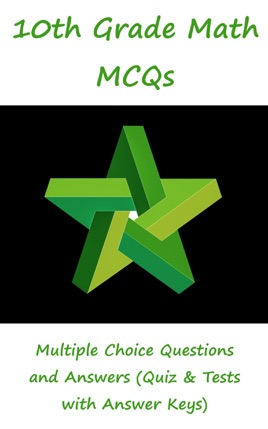• 4,99 €

## Descrizione dell’editore

"10th Grade Math MCQs: Multiple Choice Questions and Answers (Quiz & Tests with Answer Keys)" provides mock tests for competitive exams preparation. "Grade 10 Math MCQ" pdf to download helps with theoretical, conceptual, and analytical study for self-assessment, career tests. 10th grade math quizzes, a quick study guide can help to learn and practice questions for placement test preparation.
"Grade 10 Math Multiple Choice Questions and Answers (MCQs)" pdf to download is a revision guide with a collection of trivia questions to fun quiz questions and answers pdf on topics: Introduction to trigonometry, 3rd, 4th, mean and continued proportion, algebraic formulas and applications, algebraic manipulation, basic statistics, factorization, geometry, linear equations and inequalities, matrices and determinants, ratio, proportions and variations, roots of quadratic equations, sets and functions to enhance teaching and learning.
Grade 10 Math Quiz Questions and Answers pdf also covers the syllabus of many competitive papers for admission exams of different schools from math textbooks on chapters:

3rd, 4th, Mean and Continued Proportion Multiple Choice Questions: 9 MCQs. Algebraic Formulas and Applications Multiple Choice Questions: 24 MCQs. Algebraic Manipulation Multiple Choice Questions: 13 MCQs. Basic Statistics Multiple Choice Questions: 28 MCQs. Factorization Multiple Choice Questions: 7 MCQs. General Math Questions Multiple Choice Questions: 26 MCQs. Geometry Multiple Choice Questions: 42 MCQs. Introduction to Trigonometry Multiple Choice Questions: 41 MCQs. Linear Equations and Inequalities Multiple Choice Questions: 25 MCQs. Matrices and Determinants Multiple Choice Questions: 47 MCQs. Ratio, Proportions and Variations Multiple Choice Questions: 21 MCQs. Roots of Quadratic Equations Multiple Choice Questions: 24 MCQs. Sets and Functions Multiple Choice Questions: 24 MCQs.

"3rd, 4th, Mean and Continued Proportion MCQ" pdf chapter covers quiz about continued proportional, fourth proportional, mean proportional, and third proportional.
"Algebraic Formulas and Applications MCQ" pdf chapter covers quiz about algebraic expressions, math formulas, surds and surds applications.
"Basic Statistics MCQ" pdf chapter covers quiz about construction of frequency polygon, construction of histograms, frequency distribution, measures of central tendency, and measures of dispersion.
"Factorization MCQ" pdf chapter covers quiz about factorization of expressions, and math theorems.
"General Math Questions MCQ" pdf chapter covers quiz about basic concepts, circle's basic concepts, fraction, improper fraction, proper fraction, rational fraction, math theorems, parallel lines, relation between roots, and coefficients.
"Geometry MCQ" pdf chapter covers quiz about circles, radius of circle, escribed circle, cylinder, lines and angles, polygon, rectangle, and triangle.
"Introduction to Trigonometry MCQ" pdf chapter covers quiz about trigonometric identities, trigonometric ratios, area of circular sector, co terminal angles, conversion: radians and degrees, degree, measurement of angles, quadrants, radian measure of angle, reciprocal identities, relation between radians and degree, and sector of circle.
"Matrices and Determinants MCQ" pdf chapter covers quiz about introduction to matrices, types of matrices, addition and subtraction of matrices, multiplication of matrices, multiplicative inverse of matrix, and solution of simultaneous linear equations.
"Ratio, Proportions and Variations MCQ" pdf chapter covers quiz about ratios, proportion, variation, joint variation, k method, and math theorems.